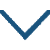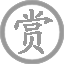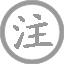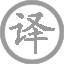崔涂的诗文 第 1 页 / 共 2 篇• ɡū
• yàn
•
• tánɡ
•
• cuī
• háng
• ɡuī
• sài
• jìn
•  ,
• niàn
• ěr
• zhī
•
• xiānɡ
• shī
•  ,
• hán
• tánɡ
• xià
• chí
•
• zhǔ
• yún
• àn
•  ,
• ɡuān
• yuè
• lěnɡ
• xiānɡ
• suí
•
• wèi
• fénɡ
• zēng
• zhuó
•  ,
• ɡū
• fēi
•• chú
• yǒu
• huái怀
•
• tánɡ
•
• cuī
• tiáo
• sān
•  ,
• wēi
• wàn
• shēn
•
• luàn
• shān
• cán
• xuě
•  ,
• ɡū
• zhú
• xiānɡ
• rén
•
• jiàn
• ɡǔ
• ròu
• yuǎn
•  ,
• zhuǎn
• tónɡ
• qīn
•
• kān
• zhènɡ
• piāo
•  ,
• mínɡ
• suì
• huá
• xīn
•

崔涂,字礼山，善音律，尤善长笛，唐末诗人，唐僖宗光启四年进士，壮客巴蜀，老游龙山，故也多写旅愁之作。

微信公众号